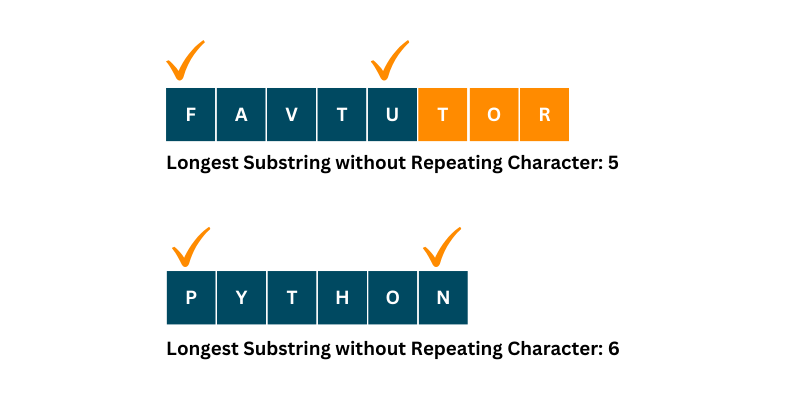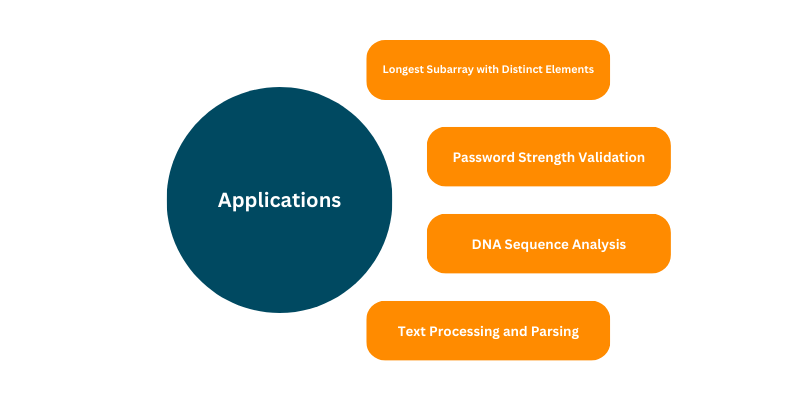# Longest Substring Without Repeating Characters in Python

In the domain of competitive programming and coding interviews, having a knack for efficiently solving complex problems is highly sought after. One prime example of such a challenge is the task of finding the longest substring without any repeated characters. The goal of this article is to delve deeply into this problem, presenting various approaches, and shedding light on the methods for determining the length of the longest substring without any character repetitions.

## Understanding the Problem

Let's begin by establishing a clear understanding of the problem. Given a string, our goal is to identify the longest substring within that string in which no character is repeated. Essentially, we are searching for the longest unique substring within the input string. We will also try to find a solution with the optimal time complexity.For instance, consider the input string "FAVTUTOR". The longest substring without repeating characters, in this case, is "FAVTU," with a length of 5. Similarly, for the word "PYTHON", it’s 6.

## Brute Force Approach

To gain a better understanding of the problem, we can start by examining a brute force solution. In this method, we generate all possible substrings of the input string and check each substring for the presence of repeating characters.

Although this approach is straightforward, it proves highly inefficient, especially for longer input strings.

Here's a simplified Python code snippet to illustrate the brute force approach:

Code:

```def longest_unique_substring(s):
max_length = 0

for i in range(len(s)):
for j in range(i, len(s)):
if len(set(s[i:j+1])) == j - i + 1:
max_length = max(max_length, j - i + 1)
longest_substring = s[i:j+1]

return max_length, longest_substring

def main():
input_string = "FAVTUTOR"
max_length, longest_substring = longest_unique_substring(input_string)
print(f"Input String: {input_string}")
print(f"Longest Substring without Repeating Characters: {longest_substring}")
print(f"Length of the Longest Substring: {max_length}")

if __name__ == "__main__":
main()
```

Output:

```Input String: FAVTUTOR
Longest Substring without Repeating Characters: FAVT
Length of the Longest Substring: 4
```

The brute force approach uses nested loops to consider all possible substrings of the input string. The outer loop (i) iterates through each character as a potential starting position, while the inner loop (j) scans through characters from the starting position to the end of the string. For each substring candidate (s[i:j+1]), it creates a set to check for repeating characters. If no repeating characters are found, the length of the candidate substring is compared to the current maximum length. The approach continues to evaluate all substrings and updates the maximum length and longest substring as it finds longer unique substrings.

### Time and Space Complexity

The time complexity of this approach is O(n^3), where n is the length of the input string. It is evident that this is not an efficient solution for practical applications. The auxiliary space complexity of this problem is O(1).

## Sliding Window Approach

To substantially improve the time complexity of our solution, we can employ the sliding window technique. The core idea is to maintain a window of characters and slide it through the input string, ensuring that no repeating characters are present within the window.

Here's a Python implementation of the sliding window approach:

Code:

```def longest_unique_substring(s):
max_length = 0
char_index = {}
start = 0
longest_substring = ""

for end in range(len(s)):
if s[end] in char_index and char_index[s[end]] >= start:
start = char_index[s[end]] + 1
char_index[s[end]] = end
if end - start + 1 > max_length:
max_length = end - start + 1
longest_substring = s[start:end+1]

return max_length, longest_substring

def main():
input_string = "FAVTUTOR"
max_length, longest_substring = longest_unique_substring(input_string)
print(f"Input String: {input_string}")
print(f"Longest Substring without Repeating Characters: {longest_substring}")
print(f"Length of the Longest Substring: {max_length}")

if __name__ == "__main__":
main()
```

Output:

```Input String: FAVTUTOR
Longest Substring without Repeating Characters: FAVT
Length of the Longest Substring: 4
```

In this code, we use a sliding window represented by the 'start' and 'end' indices, and a dictionary 'char_index' to store the last index of each character encountered.

### Time and Space Complexity

This approach results in a time complexity of O(n), where n is the length of the input string. The space complexity remains the same at O(1).

## Optimizing the Solution

While the sliding window approach is efficient, further optimization is possible. We can eliminate the need to track the index of every character encountered and instead directly update the 'start' index.

Code:

```def longest_unique_substring(s):
max_length = 0
char_index = {}
start = 0
longest_substring = ""

for end in range(len(s)):
if s[end] in char_index:
start = max(start, char_index[s[end]] + 1)
char_index[s[end]] = end
if end - start + 1 > max_length:
max_length = end - start + 1
longest_substring = s[start:end+1]

return max_length, longest_substring

def main():
input_string = "FAVTUTOR"
max_length, longest_substring = longest_unique_substring(input_string)
print(f"Input String: {input_string}")
print(f"Longest Substring without Repeating Characters: {longest_substring}")
print(f"Length of the Longest Substring: {max_length}")

if __name__ == "__main__":
main()
```

Output:

```Input String: FAVTUTOR
Longest Substring without Repeating Characters: FAVT
Length of the Longest Substring: 4
```

### Time and Space Complexity

By implementing this optimized approach, we can efficiently find the length of the longest substring without repeating characters in O(n) time. The space complexity for this problem is fixed at O(1).

## Handling Edge Cases

While the sliding window approach is powerful, it's crucial to consider edge cases and additional scenarios.

For example, what if the input string contains spaces or special characters? How can we handle Unicode characters or non-ASCII characters?

### Handling Spaces and Special Characters

When dealing with input strings that contain spaces or special characters, the sliding window approach remains effective. Spaces and special characters are treated like any other character within the string. The algorithm will correctly identify the longest substring without repeating characters, including spaces and special characters.

### Handling Unicode and Non-ASCII Characters

To handle Unicode or non-ASCII characters, you can still use the sliding window approach. Unicode characters are treated as individual characters within the input string. The algorithm works as expected by identifying the longest substring without repeating characters, irrespective of the character encoding used.

## Variations and Applications

The concept of finding the longest substring without repeating characters has various applications in computer science and programming. Here are some notable variations and use cases:### 1. Longest Subarray with Distinct Elements

In an array of numbers, finding the longest subarray with distinct elements follows a similar approach. Instead of characters, you work with integers and apply the sliding window technique to achieve the desired result.

Many websites and applications use a password strength meter that evaluates the uniqueness of characters in a password. By understanding how to find the longest substring without repeating characters, you can create more robust password strength validation algorithms.

### 3. DNA Sequence Analysis

In bioinformatics, analyzing DNA sequences often involves identifying unique subsequences or patterns within a given DNA sequence. The techniques used to find the longest substring without repeating characters can be adapted for such analyses.

### 4. Text Processing and Parsing

Natural language processing and text analysis frequently require the identification of unique phrases or words within a text. The algorithms developed for this problem can be instrumental in these applications.

## Real-World Examples

To demonstrate the practical application of finding the longest substring without repeating characters, let's consider two real-world examples.

### Example 1: Password Strength Checker

Imagine you are building a registration system for a website, and you want to ensure that users create strong and unique passwords. By employing the longest unique substring algorithm, you can check if the password contains a sufficiently long substring without repeating characters, making it more secure.

### Example 2: Language Translation

In a machine translation system, it's essential to identify unique words or phrases in the source language to improve translation accuracy. By utilizing the techniques discussed in this article, you can preprocess the text and extract unique segments to enhance the translation process.

## Conclusion

In this article, we dug into the intricate problem of finding the longest substring without repeating characters in Python. We began by discussing the brute force approach and highlighted its inefficiency, paving the way for the sliding window technique. The sliding window approach, along with optimizations, provided an efficient solution to the problem, with a time complexity of O(n).

### FavTutor - 24x7 Live Coding Help from Expert Tutors!